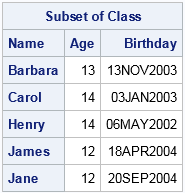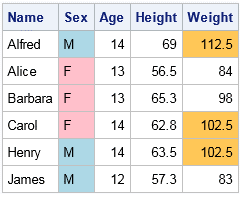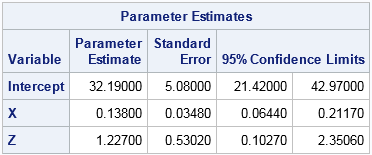One of the advantages of the new mixed-type tables in SAS/IML 14.2 (released with SAS 9.4m4) is the greatly enhanced printing functionality. You can control which rows and columns are printed, specify formats for individual columns, and even use templates to completely customize how tables are printed. Printing a table is accomplished by using the TablePrint subroutine, which has many more options than the well-known SAS/IML PRINT statement for matrices.

### The TablePrint subroutine

The TablePrint subroutine provides many options that give you control over the output:

• The FIRSTOBS= and NUMOBS= options specify the range of observations to print.
• The ID= option specifies a variable to use for the row header on the left side of the table.
• The VAR= option specifies the columns (and the column order) for the table.
• The LABEL= option specifies a spanning header for the table.

For example, the following DATA step creates random birthdays for the students in the Sashelp.Class data set. The Birthday variable is formatted with the DATE9. format. The data is then read into a SAS/IML table by using the TableCreateFromDataSet function. Finally, the TablePrint subroutine prints a customize portion of the table:

```data class; set sashelp.class; Birthday = '03APR2017'd - age*365 - floor(365*uniform(1)); /* create birthday */ format Birthday DATE9.; run;   proc iml; tClass = TableCreateFromDataSet("Class"); /* read data into table */ run TablePrint(tClass) firstobs=3 numobs=5 var={"Age" "Birthday"} ID="Name" label="Subset of Class";```Notice that the table contains both numeric and character columns. Furthermore, the numeric columns have different formats. The TablePrint subroutine has some distinct advantages over the traditional PRINT statement in SAS/IML:

• The TablePrint subroutine supports an easy way to display a range of observations. When you use the PRINT statement for multiple vectors, you have to use row subscripts in each vector, such as PRINT (X[3:8,]) (Y[3:8,]);
• The TablePrint subroutine supports printing any columns in any order. When you use the PRINT statement on a matrix, you have to use column subscripts to change the order of the matrix columns: PRINT (X[, {2 3 1}]);
• The PRINT statement supports the ROWNAME= option (for specifying row headers), the COLNAME= option (for specifying column headers), and the LABEL= option. Those options are easy to work with when you print a single matrix. However, you can't store mixed-type data in a matrix and those options are less convenient when you print a set of vectors.

### Advanced printing of SAS/IML tablesThe SAS/IML documentation has several sections of documentation devoted to advanced printing of SAS/IML tables. For example, you can use the TablePrint subroutine to do the following:

• Use a custom template to display a table.
• Specify values for dynamic variables in templates.
• Use an existing ODS template to format and display data that are in SAS/IML.

The documentation contains an example of "traffic lighting," where the colors of cells depend on the value in the cells, as shown to the right.

For statistical programmers, the ability to use ODS templates means that output from PROC IML can look the same as output from some other SAS procedue. For example, suppose that you have a table that contains parameter estimates for a linear regression. The following example prints that table by using the same ODS template that PROC REG uses, which is the Stat.Reg.ParameterEstimates template:

```proc iml; vars = {"Intercept", X, Z}; stats = {32.19 5.08 21.42 42.97, 0.138 0.0348 0.0644 0.2117, 1.227 0.5302 0.1027 2.3506 }; tbl = TableCreate("Variable", vars); call TableAddVar(tbl, {"Estimate" "StdErr" "LowerCL" "UpperCL"}, stats);   Confidence=95; call TablePrint(tbl) template="Stat.Reg.ParameterEstimates" dynamic={Confidence};```This example works because the column names in the SAS/IML table match the names that are expected by the Stat.REG.ParameterEstimates template. The DYNAMIC= option specifies a dynamic variable (Confidence) that the template requires. See the documentation for further details.

### Summary

In summary, the TablePrint subroutine in SAS/IML gives programmers control over many options for printing tables of data and statistics. For complex layouts, you can use an existing ODS template or create your own template to customize virtually every aspect of your tabular displays. For more information about SAS/IML tables, see the SAS Global Forum paper "More Than Matrices" (Wicklin, 2017).

ShareDistinguished Researcher in Computational Statistics

Rick Wicklin, PhD, is a distinguished researcher in computational statistics at SAS and is a principal developer of SAS/IML software. His areas of expertise include computational statistics, simulation, statistical graphics, and modern methods in statistical data analysis. Rick is author of the books Statistical Programming with SAS/IML Software and Simulating Data with SAS.

1.Paige Miller on

•